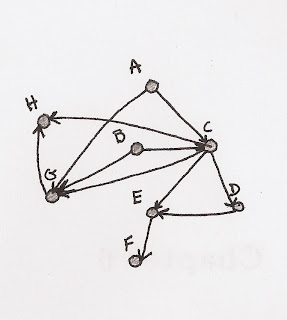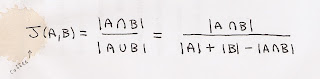Tuesday, May 3, 2011

Structural Similarity With Apache Pig

A while ago I posted this about computing the jaccard similarity (otherwise known as the structural similarity) of nodes in a network graph. Looking back on it over the past few days I realize there are some areas for serious improvement. Actually, it's just completely wrong. The approach is broken. So, I'm going to revisit the algorithm again, in more detail, and write a vastly improved Pig script for computing the structural similarity of vertices in a network graph.

The graph

Of course, before you can do a whole lot of anything, you've got to have a graph to work with. To keep things dead simple (and breaking from what I normally do) I'm going to draw an arbitrary graph (and by draw I mean draw). Here it is:This can be represented as a tab-separated-values set of adjacency pairs in a file called 'graph.tsv':

$: cat graph.tsvA CB CC DC EE FC HG HC GD EA GB G I don't think it gets any simpler. The Measure Now, the idea here is to compute the similarity between nodes, eg similarity(A,B). There's already a well defined measure for this called the jaccard similarity. The key take away is to notice that:The Pig This can be broken into a set of Pig operations quite easily actually: load data Remember, I saved the data into a file called 'graph.tsv'. So: edges = LOAD 'graph.tsv' AS (v1:chararray, v2:chararray);edges_dup = LOAD 'graph.tsv' AS (v1:chararray, v2:chararray); It is necessary, but still a hack, to load the data twice at the moment. This is because self-intersections (which I'll be doing in a moment) don't work with Pig 0.8 at the moment. augment with sizes Now I need the 'size' of each of the sets. In this case the 'sets' are the list of nodes each node links to. So, all I really have to do is calculate the number of outgoing links: grouped_edges = GROUP edges BY v1;aug_edges = FOREACH grouped_edges GENERATE FLATTEN(edges) AS (v1, v2), COUNT(edges) AS v1_out;grouped_dups = GROUP edges_dup BY v1;aug_dups = FOREACH grouped_dups GENERATE FLATTEN(edges_dup) AS (v1, v2), COUNT(edges_dup) AS v1_out; Again, if self-intersections worked, the operation of counting the outgoing links would only have to happen once. Now I've got a handle on the |A| and |B| parts of the equation above. intersection Next I'm going to compute the intersection using a Pig join: edges_joined = JOIN aug_edges BY v2, aug_dups BY v2;intersection = FOREACH edges_joined { -- -- results in: -- (X, Y, |X| + |Y|) -- added_size = aug_edges::v1_out + aug_dups::v1_out; GENERATE aug_edges::v1 AS v1, aug_dups::v1 AS v2, added_size AS added_size ; }; Notice I'm adding the individual set sizes. This is to come up the |A| + |B| portion of the denominator in the jaccard index. intersection sizes In order to compute the size of the intersection I've got to use a Pig GROUP and collect all the elements that matched on the JOIN: intersect_grp = GROUP intersection BY (v1, v2);intersect_sizes = FOREACH intersect_grp { -- -- results in: -- (X, Y, |X /\ Y|, |X| + |Y|) -- intersection_size = (double)COUNT(intersection); GENERATE FLATTEN(group) AS (v1, v2), intersection_size AS intersection_size, MAX(intersection.added_size) AS added_size -- hack, we only need this one time ; }; There's a hack in there. The reason is that 'intersection.added_size' is a Pig data bag containing some number of identical tuples (equal to the intersection size). Each of these tuples is the 'added_size' from the previous step. Using MAX is just a convenient way of pulling out only one of them. similarity And finally, I have all the pieces in place to compute the similarities: similarities = FOREACH intersect_sizes { -- -- results in: -- (X, Y, |X /\ Y|/|X U Y|) -- similarity = (double)intersection_size/((double)added_size-(double)intersection_size); GENERATE v1 AS v1, v2 AS v2, similarity AS similarity ; }; That'll do it. Here's the full script for completeness: edges = LOAD '$GRAPH' AS (v1:chararray, v2:chararray);edges_dup = LOAD '$GRAPH' AS (v1:chararray, v2:chararray);---- Augment the edges with the sizes of their outgoing adjacency lists. Note that-- if a self join was possible we would only have to do this once.--grouped_edges = GROUP edges BY v1;aug_edges = FOREACH grouped_edges GENERATE FLATTEN(edges) AS (v1, v2), COUNT(edges) AS v1_out;grouped_dups = GROUP edges_dup BY v1;aug_dups = FOREACH grouped_dups GENERATE FLATTEN(edges_dup) AS (v1, v2), COUNT(edges_dup) AS v1_out;---- Compute the sizes of the intersections of outgoing adjacency lists--edges_joined = JOIN aug_edges BY v2, aug_dups BY v2;intersection = FOREACH edges_joined { -- -- results in: -- (X, Y, |X| + |Y|) -- added_size = aug_edges::v1_out + aug_dups::v1_out; GENERATE aug_edges::v1 AS v1, aug_dups::v1 AS v2, added_size AS added_size ; };intersect_grp = GROUP intersection BY (v1, v2);intersect_sizes = FOREACH intersect_grp { -- -- results in: -- (X, Y, |X /\ Y|, |X| + |Y|) -- intersection_size = (double)COUNT(intersection); GENERATE FLATTEN(group) AS (v1, v2), intersection_size AS intersection_size, MAX(intersection.added_size) AS added_size -- hack, we only need this one time ; };similarities = FOREACH intersect_sizes { -- -- results in: -- (X, Y, |X /\ Y|/|X U Y|) -- similarity = (double)intersection_size/((double)added_size-(double)intersection_size); GENERATE v1 AS v1, v2 AS v2, similarity AS similarity ; };DUMP similarities; Results Run it: $: pig -x local jaccard.pig...snip...(A,A,1.0)(A,B,1.0)(A,C,0.2)(B,A,1.0)(B,B,1.0)(B,C,0.2)(C,A,0.2)(C,B,0.2)(C,C,1.0)(C,D,0.25)(C,G,0.25)(D,C,0.25)(D,D,1.0)(E,E,1.0)(G,C,0.25)(G,G,1.0)

And it's done! Hurray. Now, go make a recommender system or something.